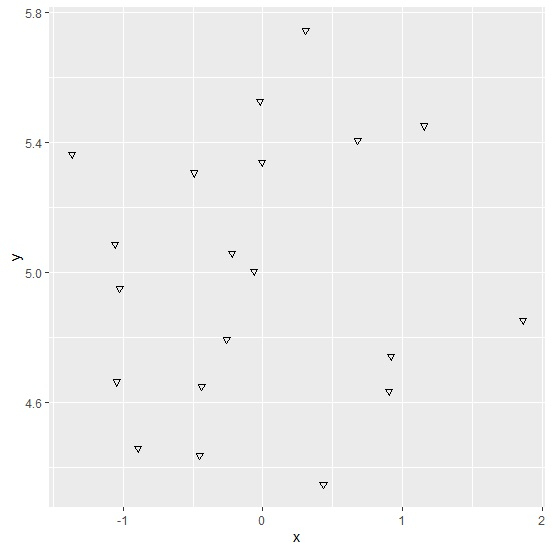# How to change the default type of points for scatterplot using ggplot2 in R?

To change the defaults for ggplot2 geoms, we need to use update_geom_defaults function. If we want to change the shape of points for scatterplot, we can use the below syntax −

update_geom_defaults("point",list(shape=”point_shape_number”))

The point shape number ranges from 0 to 25. We can change that according to our need.

Consider the below data frame −

## Example

Live Demo

x<-rnorm(20)
y<-rnorm(20,5,0.32)
df<-data.frame(x,y)
df

## Output

        x           y
1  -0.005184157  5.338089
2  -1.044878945  4.662460
3  1.154191529   5.450373
4  -0.437975797  4.649176
5  -0.220793320  5.057522
6  -0.062619288  5.003642
7  -1.023676613  4.949002
8  0.902098608   4.632925
9  -0.259247125  4.792074
10 0.305164402   5.745798
11 -0.891792853  4.458326
12 -0.451765777  4.435208
13 -0.018278677  5.525500
14 0.917192389   4.740232
15 -1.366201294  5.363222
16 -0.491698757  5.305723
17 0.680064934   5.406010
18 -1.058529703  5.084462
19 1.862063812   4.851102
20 0.433153374   4.346126

Loading ggplot2 package and creating a scatterplot between x and y −

## Example

library(ggplot2)
ggplot(df,aes(x,y))+geom_point()

## OutputUpdating point type for scatterplot of ggplot2 package and creating the plot −

## Example

update_geom_defaults("point",list(shape=6))
ggplot(df,aes(x,y))+geom_point()

## Output